sk|cz|

# Statistics

1. Explain following concepts:

• distribution characteristics (average)
• variability characteristics
Solution:

## Distribution characteristics

a) Arithmetic mean: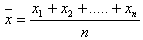b) Geometric mean: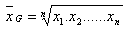c) Harmonic mean: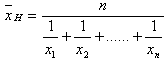d) Mode mod(x) is the value that occurs the most frequently in a data set or a probability distribution.

e) Median med(x) is:
• the middle value of a data set sorted by the value containing odd number of elements
• aritmethmetic mean of the two middle values of a data set sorted by the value containing even number of elements

## Variability characteristics

a) Range

R = xmax - xmin

b) Variance (dispersion)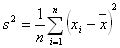c) Standard deviation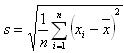2. Following values were measured in the lab (in milimeters):
{302;310;312;310;313;318;305;309;310;309}

Determine the arithmetic mean, geometric mean, geometrický priemer, mode and median.
Solution:

Sorted values:
{302;305;309;309;310;310;310;312;313;318}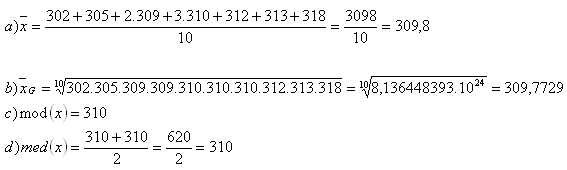3.A car hit an average speed of v1 = 20 kmph in the first half of the journey, and hit an average speed of v2 = 80 kmph in the second half of the journey. Determine the average speed of the car.

Solution:

The car moved in different speeds, therefore it passed each half in different time.
The average speed equals to the harmonic mean of v1 and v2.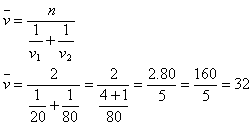The average speed of the car was 32 kmph.

4.Following values were measured to determine the weight of a cog wheel. Find out the interval which contains the real weight value with a probability of 68%.

M = {324;330;327;319;334;304}
Solution: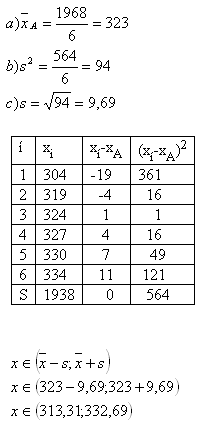5. Hounters A and B compete in target shooting. Who was more accurate and won the competition?

Results:
A = {9;8;8;8;7}
B = {10;10;8;7;5}
Solution:

Hunter A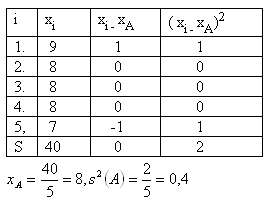Hunter B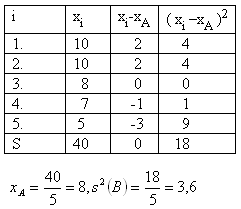Variance of hunter A equals s2(A) = 0,4 , variance of hunter B equals s2(B) = 3,6. Stands s2(A) < s2(B).
Hunter A won the competition.